#Function Repository Resource:

# HappyNumberQ

Test whether an integer is a happy number

Contributed by: Sander Huisman
 ResourceFunction["HappyNumberQ"][n] tests whether repeatedly summing up the square of the digits of n reaches 1. ResourceFunction["HappyNumberQ"][n,b] considers the integer digits of n in base b. ResourceFunction["HappyNumberQ"][n,b,p] raises the digits to the power p.

## Details and Options

The base b has to be at least 2.
The power p has to be at least 1.

## Examples

### Basic Examples

Test whether 19 is a happy number:

 In:=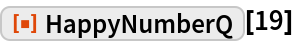Out=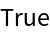Test whether 1579 is a happy number based on its digits in base 7:

 In:=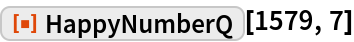Out=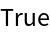### Scope

Cube the numbers rather than squaring them:

 In:=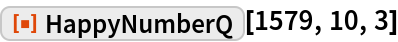Out=### Properties and Relations

In base 2, all numbers are happy:

 In:=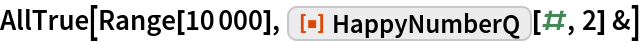Out=### Possible Issues

 In:=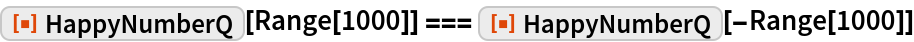Out=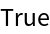Checking for higher powers can take a lot more time:

 In:=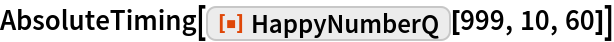Out=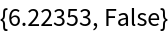### Neat Examples

Find numbers that are happy in many bases:

 In:=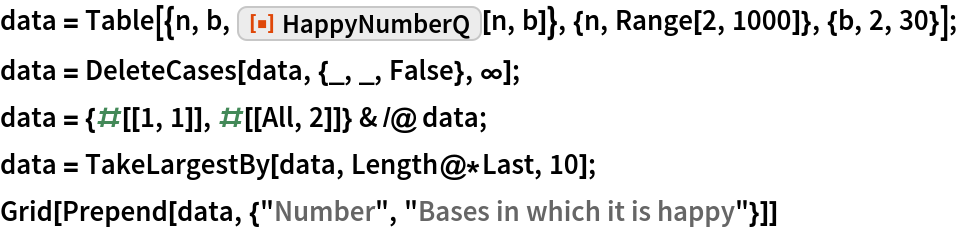Out=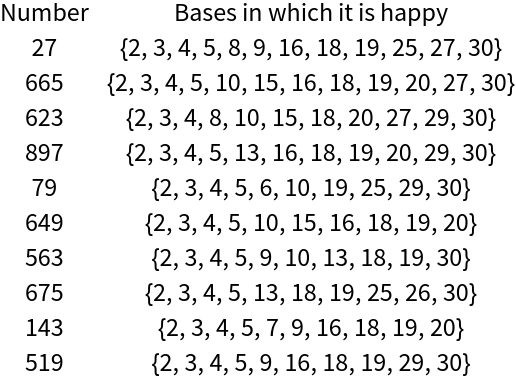Find happy numbers that are also prime:

 In:=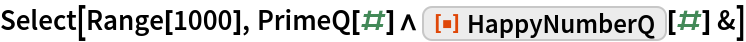Out=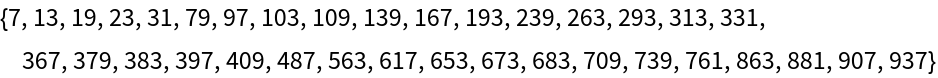## Requirements

Wolfram Language 11.3 (March 2018) or above